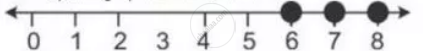Share

# Solve and Graph the Solution Set Of: 2x – 9 ≤ 7 and 3x + 9 > 25, X ∈ I - Mathematics

Course

#### Question

Solve and graph the solution set of:

2x – 9 ≤ 7 and 3x + 9 > 25, x ∈ I

#### Solution

2x - 9 <= 7 and 3x + 9 > 25

2x  <= 16  and 3x > 16

x <= 8 and x > 5 1/3

∴ Solution set = {5 1/3 < x <= 8, x in I} = {6, 7, 8}

The required graph on number line isIs there an error in this question or solution?